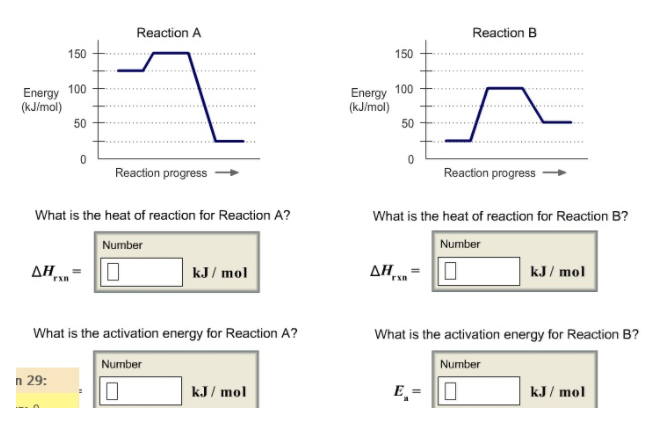# Problem: What is the heat of reaction for Reaction A? What is the heat of reaction for Reaction B? What is the activation energy for Reaction A? What is the activation energy for Reaction B?

###### FREE Expert Solution
82% (123 ratings)
###### FREE Expert Solution
82% (123 ratings)###### Problem Details

What is the heat of reaction for Reaction A? What is the heat of reaction for Reaction B? What is the activation energy for Reaction A? What is the activation energy for Reaction B?What scientific concept do you need to know in order to solve this problem?

Our tutors have indicated that to solve this problem you will need to apply the Energy Diagram concept. If you need more Energy Diagram practice, you can also practice Energy Diagram practice problems.

What is the difficulty of this problem?

Our tutors rated the difficulty ofWhat is the heat of reaction for Reaction A? What is the hea...as medium difficulty.

How long does this problem take to solve?

Our expert Chemistry tutor, Dasha took 5 minutes and 28 seconds to solve this problem. You can follow their steps in the video explanation above.

What professor is this problem relevant for?

Based on our data, we think this problem is relevant for Professor Bussey's class at UCSD.